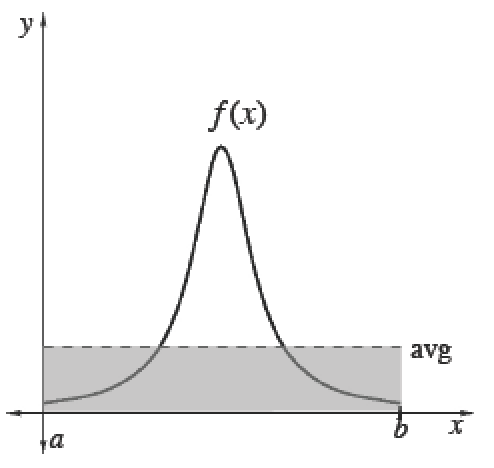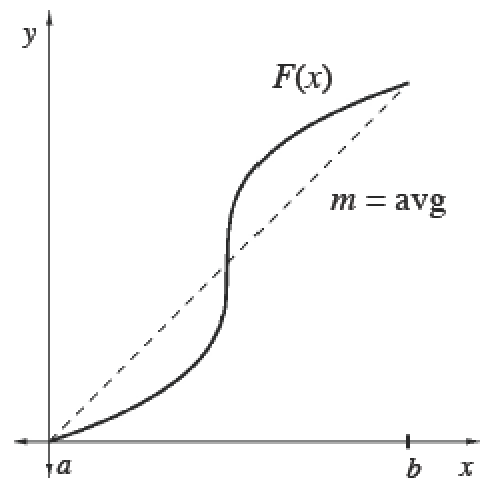### Home > APCALC > Chapter 8 > Lesson 8.2.2 > Problem8-84

8-84.

Multiple Choice: The mean value of the function $f ( x ) = x \sqrt { 1 - x ^ { 2 } }$ over the interval $0 ≤ x ≤ 1$ is:

1. $\frac { 1 } { 3 }$

1. $\frac { 1 } { 6 }$

1. $\frac { 2 } { 3 }$

1. $\frac { 1 } { 2 }$

1. $\frac { 1 } { 4 }$

Think about the information you are given and think about what you want to know. Mean value of WHAT?
Will you integrate and then divide? Or will you compute the slope of the secant line?

Average (Mean) Values

 To calculate the mean (average) value of a finite set of items, add up the values of items and divide by the number of items.Integrals help us add over a continuous interval. Therefore, for any continuous function $f$ :$\frac{\int_a^bf(x)dx}{b-a}=$ mean value of $f$ over $[a, b]$Since $\int _ { a } ^ { b } f ( x ) d x$, we can also calculate the average value of any function $f$ using its antiderivative $F$. Its average slope gives the average rate of change of $F$, which is the same as the average value of $f.$$\frac{\int_a^bf(x)dx}{b-a}=\frac { F ( b ) - F ( a ) } { b - a }=$ mean rate of change of $F ′$ over $[a, b]$